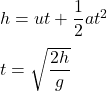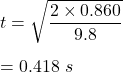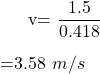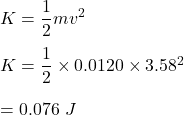## A student at another university repeats the experiment you did in lab. Her target ball is 0.860 m above the floor when it is in the target h

Question

A student at another university repeats the experiment you did in lab. Her target ball is 0.860 m above the floor when it is in the target holder and the steel ball she uses has a mass of 0.0120 kg. She finds that the target ball travels a distance of 1.50 m after it is struck. Assume g = 9.80 m/s2. What is the kinetic energy (in joules) of the target ball just after it is struck?

in progress 0
7 months 2021-07-17T16:27:37+00:00 1 Answers 0 views 0

K = 0.076 J

Explanation:

The height of the target, h = 0.860  m

The mass of the steel ball, m = 0.0120 kg

Distance moved, d = 1.50 m

We need to find the kinetic energy (in joules) of the target ball just after it is struck. Let t is the time taken by the ball to reach the ground.Put all the values,The velocity of the ball is :The kinetic energy of the ball is :So, the required kinetic energy is 0.076 J.